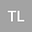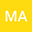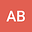Blow-up of result in a nonlinear wave equation with delay and source term
•••• Tayeb Lakroumbe,
• Mama Abdelli,
• Abderrahmane Beniani
Tayeb Lakroumbe
University of Djillali Liabes Faculty of Exact Sciences

Corresponding Author:tayeblakroumbe@yahoo.fr

Author ProfileMama Abdelli
University of Djillali Liabes Sidi Bel Abbes
Author ProfileAbderrahmane Beniani
Center University of Belhadj Bouchaib -B.P. 284 RP, Ain Temouchent
Author Profile## Abstract

In this paper we consider the initial boundary value problem for a nonlinear damping and a delay term of the form:

\begin{equation} |u_{t}|^{l}u_{tt}-\Delta u(x,t)-\Delta u_{tt}+\mu_{1}|u_{t}|^{m-2}u_{t}\\ +\mu_{2}|u_{t}(t-\tau)|^{m-2}u_{t}(t-\tau)=b|u|^{p-2}u,\nonumber \\ \end{equation}

with initial conditions and Dirichlet boundary conditions. Under appropriate conditions on $$\mu_{1},\,\ \mu_{2}$$, we prove that there are solutions with negative initial energy that blow-up finite time if $$p\geq\max\{l+2,m\}$$.

Dec 2021Published in The interdisciplinary journal of Discontinuity, Nonlinearity, and Complexity volume 10 issue 4 on pages 733-741. 10.5890/DNC.2021.12.012